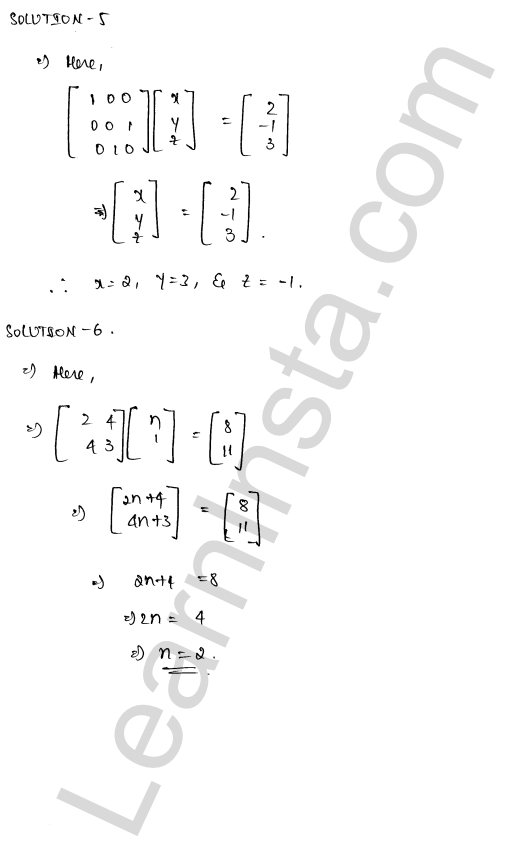RD Sharma Class 12 Solutions Chapter 8 Solution of Simultaneous Linear Equations Ex 8.1 are part of RD Sharma Class 12 Solutions. Here we have given RD Sharma Class 12 Solutions Chapter 8 Solution of Simultaneous Linear Equations Ex 8.1.

Here you can get free RD Sharma Solutions for Class 12 Maths of Chapter 8 Solution of Simultaneous Linear Equations Exercise 8.1. All RD Sharma Book Solutions are given here exercise wise for Solution of Simultaneous Linear Equations. RD Sharma Solutions are helpful in the preparation of several school level, graduate and undergraduate level competitive exams. Practicing questions from RD Sharma Mathematics Solutions for Class 12 Chapter 8 Solution of Simultaneous Linear Equations is proven to enhance your math skills.

 Class: 12th Class Chapter: Chapter 8 Name: Solution of Simultaneous Linear Equations Exercise: Exercise 8.1

RD Sharma Class 12 Solutions Chapter 8 Solution of Simultaneous Linear Equations VSAQWe hope the RD Sharma Class 12 Solutions Chapter 8 Solution of Simultaneous Linear Equations VSAQ, help you. If you have any query regarding RD Sharma Class 12 Solutions Chapter 8 Solution of Simultaneous Linear Equations VSAQ, drop a comment below and we will get back to you at the earliest.